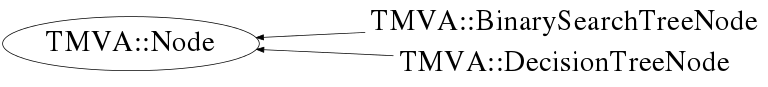# class TMVA::Node

```
Node for the BinarySearch or Decision Trees.

For the binary search tree, it basically consists of the EVENT, and
pointers to the parent and daughters

In case of the Decision Tree, it specifies parent and daughters, as
well as "which variable is used" in the selection of this node,
including the respective cut value.

```

## Function Members (Methods)

This is an abstract class, constructors will not be documented.
Look at the header to check for available constructors.

public:
 virtual ~Node() virtual void AddAttributesToNode(void* node) const virtual void AddContentToNode(stringstream& s) const void* AddXMLTo(void* parent) const static TClass* Class() Int_t CountMeAndAllDaughters() const virtual TMVA::Node* CreateNode() const int GetCount() UInt_t GetDepth() const virtual TMVA::Node* GetLeft() const virtual TMVA::Node* GetParent() const virtual TMVA::BinaryTree* GetParentTree() const char GetPos() const virtual TMVA::Node* GetRight() const virtual Bool_t GoesLeft(const TMVA::Event&) const virtual Bool_t GoesRight(const TMVA::Event&) const virtual TClass* IsA() const TMVA::Node Node() TMVA::Node Node(const TMVA::Node& n) TMVA::Node Node(TMVA::Node* p, char pos) TMVA::Node& operator=(const TMVA::Node&) virtual void Print(ostream& os) const virtual void PrintRec(ostream& os) const virtual void ReadAttributes(void* node, UInt_t tmva_Version_Code = 262656) virtual void ReadContent(stringstream& s) virtual Bool_t ReadDataRecord(istream&, UInt_t tmva_Version_Code = 262656) void ReadXML(void* node, UInt_t tmva_Version_Code = 262656) void SetDepth(UInt_t d) virtual void SetLeft(TMVA::Node* l) virtual void SetParent(TMVA::Node* p) virtual void SetParentTree(TMVA::BinaryTree* t) void SetPos(char s) virtual void SetRight(TMVA::Node* r) virtual void ShowMembers(TMemberInspector& insp) const virtual void Streamer(TBuffer&) void StreamerNVirtual(TBuffer& ClassDef_StreamerNVirtual_b)

## Data Members

protected:
 UInt_t fDepth depth of the node within the tree (seen from root node) TMVA::Node* fLeft pointers to the two "daughter" nodes TMVA::Node* fParent the previous (parent) node TMVA::BinaryTree* fParentTree pointer to the parent tree to which the Node belongs char fPos position, i.e. it is a left (l) or right (r) daughter TMVA::Node* fRight pointers to the two "daughter" nodes
private:
 static Int_t fgCount counter of all nodes present.. for debug.. to spot memory leaks...

## Class Charts## Function documentation

Node()
``` default constructor
```
Node(TMVA::Node* p, char pos)
``` constructor of a daughter node as a daughter of 'p'
```
Node(const TMVA::Node& n)
``` copy constructor, make sure you don't just copy the poiter to the node, but
that the parents/daugthers are initialized to 0 (and set by the copy
constructors of the derived classes
```
~Node()
``` node destructor
```
int GetCount()
``` retuns the global number of instantiated nodes
```
Int_t CountMeAndAllDaughters() const
```recursively go through the part of the tree below this node and count all daughters
```
``` add attributes to XML
```
void ReadXML(void* node, UInt_t tmva_Version_Code = 262656)
``` read attributes from XML
```
Node* CreateNode() const
Bool_t GoesRight(const TMVA::Event& ) const
``` test event if i{ decends the tree at this node to the right
```
Bool_t GoesLeft(const TMVA::Event& ) const
``` test event if it decends the tree at this node to the left
```
Node* GetLeft() const
``` test event if it is equal to the event that "makes the node" (just for the "search tree"
return pointer to the left/right daughter or parent node
```
`{ return fLeft; }`
Node* GetRight() const
`{ return fRight; }`
Node* GetParent() const
`{ return fParent; }`
void SetLeft(TMVA::Node* l)
``` set pointer to the left/right daughter or parent node
```
`{ fLeft = l;}`
void SetRight(TMVA::Node* r)
`{ fRight = r;}`
void SetParent(TMVA::Node* p)
`{ fParent = p;}`
void Print(ostream& os) const
``` printout of the node
```
void PrintRec(ostream& os) const
``` recursive printout of the node and it daughters
```
void SetDepth(UInt_t d)
``` Set depth, layer of the where the node is within the tree, seen from the top (root)
```
`{fDepth=d;}`
UInt_t GetDepth() const
``` Return depth, layer of the where the node is within the tree, seen from the top (root)
```
`{return fDepth;}`
void SetPos(char s)
``` set node position, i.e, the node is a left (l) or right (r) daugther
```
`{fPos=s;}`
char GetPos() const
``` Return the node position, i.e, the node is a left (l) or right (r) daugther
```
`{return fPos;}`
TMVA::BinaryTree* GetParentTree() const
``` Return the pointer to the Parent tree to which the Node belongs
```
`{return fParentTree;}`
void SetParentTree(TMVA::BinaryTree* t)
``` set the pointer to the Parent Tree to which the Node belongs
```
`{fParentTree = t;}`
Bool_t ReadDataRecord(istream& , UInt_t tmva_Version_Code = 262656)
void ReadAttributes(void* node, UInt_t tmva_Version_Code = 262656)